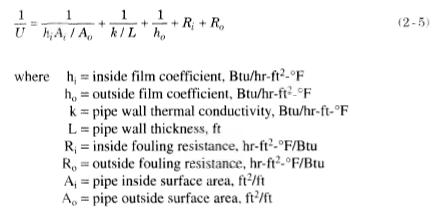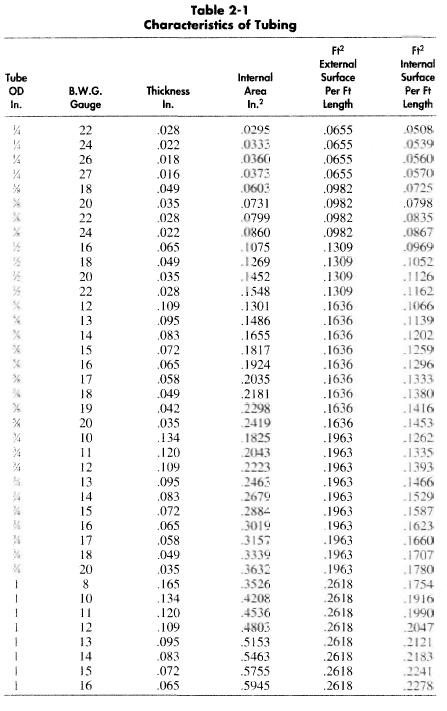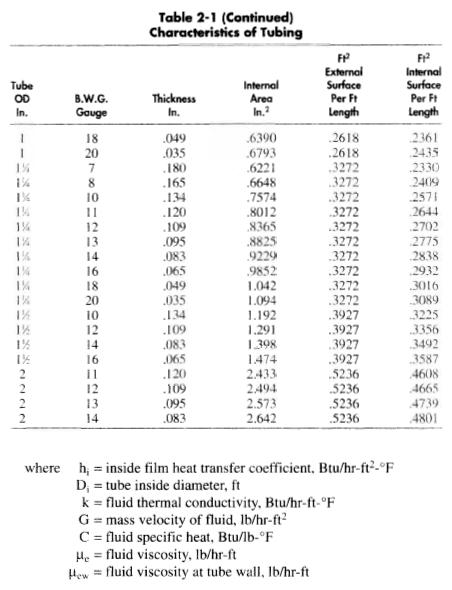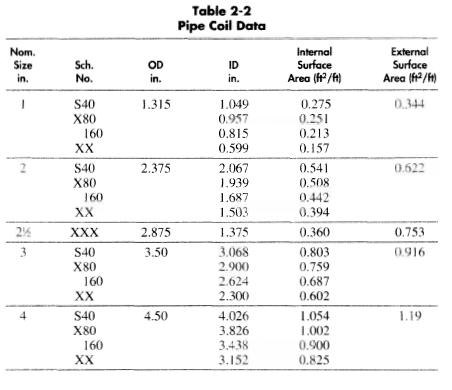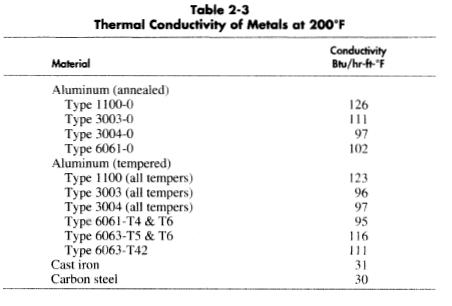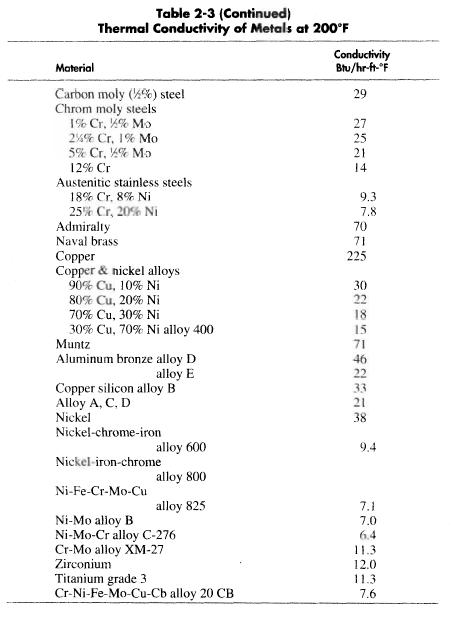﻿ Overall Heat Transfer Coefficient | Oil & Gas Process Engineering

## Overall Heat Transfer Coefficient

The overall heat transfer coefficient is a combination of the internal film coefficient, the tube wall thermal conductivity and thickness, the external film coefficient, and fouling factors. That is, in order for the energy to be transferred through the wall of the tube it has to pass through a film sitting on the inside wall of the tube. That film produces a resistance to the heat transfer, which is represented by the inside film coefficient for this convective heat transfer. It then must pass through the wall of the tube by a conduction process which is controlled by the tubewall’s thermal conductivity and tube-wall thickness. The transfer of heat from the outside wall of the tube to the bulk of the fluid outside is again a convective process. It is controlled by the outide film coefficient. All of these resistances are added in series, similar to a series of electrical resistance, to produce an overall resistance. The heat transfer coefficient is similar to the electrical conductance, and its reciprocal is the resistance.

Therefore, the following equation is used to determine the overall heat transfer coefficient for use in Equation 2-3.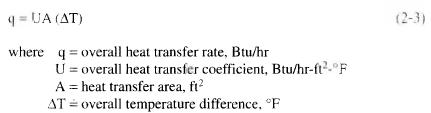Rj and R0 are fouling factors. Fouling factors are normally included to allow for the added resistance to heat flow resulting from dirt, scale, or corrosion on the tube walls. The sum of these fouling factors is normally taken to be 0.003 hr-ft2-°F/Btu, although this value can vary widely with the specific service.

Equation 2-5 gives a value for “U” based on the outside surface area of the tube, and therefore the area used in Equation 2-3 must also be the tube outside surface area. Note that Equation 2-5 is based on two fluids exchanging heat energy through a solid divider. If additional heat exchange steps are involved, such as for finned tubes or insulation, then additional terms must be added to the right side of Equation 2-5. Tables 2-1 and 2-2 have basic tube and coil properties for use in Equation 2-5 and Table 2-3 lists the conductivity of different metals.